﻿ 基于改进随机森林的肝硬化诊断预测研究 Diagnostic Prediction of Liver Cirrhosis Based on Improved Random Forest

Computer Science and Application
Vol. 09  No. 10 ( 2019 ), Article ID: 32642 , 11 pages
10.12677/CSA.2019.910216

Diagnostic Prediction of Liver Cirrhosis Based on Improved Random Forest

Jiaxing Liu, Honglie Zhang, Yanju Liu*, Huiyu Zhang, Yanzhong Liu

School of Computer and Control Engineering, Qiqihar University, Qiqihar Heilongjiang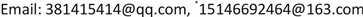Received: Oct. 2nd, 2019; accepted: Oct. 17th, 2019; published: Oct. 24th, 2019ABSTRACT

Machine learning is widely applied in the field of medical diagnosis currently. Based on the improved random forest algorithm, a prediction method for liver cirrhosis diagnosis is proposed, in which the patients’ data with liver cirrhosis indicators is analyzed and processed by means of the large amount of data obtained by patients for each examination and liver cirrhosis indicators. The method of the paper has improved the traditional diagnosis technology, adopted the random forest algorithm, used its random factor to control the characteristics of data diversity, and introduced the depth limit index. And it has improved the judgment and recognition ability of the data, and enhanced the prediction accuracy. In this paper, the data set composed of anthropometrics is used for experiments. The results show that the prediction accuracy of this method is over 90%.

Keywords:Diagnosis of Liver Cirrhosis, Improved Random Forest Algorithm, Depth Limitation, Data Prediction1. 引言

2. 随机森林Random Forest

2.1. Bagging

Bagging是一种通过使用其他数据来控制方差从而改进预测任务的方法。它通过从输入数据中随机选择n个样本来工作。样本大小与输入数据大小相同。但是，这n个样本使用替换策略，因此选择样本的

2.2. Random Forest构建过程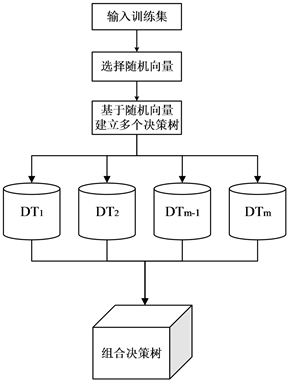Figure 1. Random forest algorithm block diagram

3. 增加视图并减小深度的随机森林

$f\left(X\right)=\frac{1}{J}\underset{j=1}{\overset{J}{\sum }}{h}_{j}\left(x\right)$ (1)

$f\left(X\right)=\mathrm{arg}ma{x}_{y\in \alpha }\underset{j=1}{\overset{J}{\sum }}I\left(y={h}_{j}\left(x\right)\right)$ (2)

$I\left(Y=f\left(x\right)\right)=\left\{\begin{array}{l}1\text{ }\text{if}\text{ }Y\text{ }\text{is}\text{ }\text{equal}\text{to}\text{ }f\left(X\right)\\ 0\text{ }\text{otherwise}\end{array}$ (3)

Step1For l = 1 to特征的数量

Step2For j = 1 to J

Step3从训练集D中提取一个大小为n的Dj作为引导子样本

Step4使用引导子样本Dj作为训练数据，使用Depth Bounded Binary Recursive Partitioning拟合树

Step5从单个节点的所有观察值开始

Step6对于深度小于l的每个未分割节点，以递归方式重复以下步骤：

Step7从p个可用预测变量中随机选择m个预测变量

Step8在步骤(6)的m个预测变量上找到所有二进制分裂中的最佳二进制分裂

Step9使用步骤(7)，将节点拆分为两个后代节点

Step10结束Forj循环

Step11在新点x进行预测，使用公式(2)计算f(x)。hj(x)则使用第j个树预测x处的响应变量

Step12计算RF的预测精度，直至达到终止条件，停止FOR循环

DBRF算法中的第四步使用深度有界二进制递归分区(Depth Bounded Binary Recursive Partitioning)算法(算法2)来构造深度有界树。DBBRP算法类似于原始的二进制递归分区算法 ，具体过程如下：

Step1从单个节点中的所有观测值 $\left({x}_{1},{y}_{1}\right),\cdots ,\left({x}_{N},{y}_{N}\right)$ 开始

Step2对于深度低于l的每个未分割节点，以递归方式重复以下步骤：

Step3在所有p个预测变量上的所有二进制分裂中找到最佳二进制分割

Step4使用最佳拆分(步骤3)将节点拆分为两个后代节点

Step5对于x处的预测，将x向下传递到树中，直到它落在终端节点中

No 1. 每棵树的RF学习速度增加。

No 2. 存储在存储器中的树的大小要比标准RF树小得多。

No 3. 评估更多的视图可以减少错过重要特征的机率，从而提高准确性和可靠性分类器。

4. 实验结果及分析

4.1. 样本组成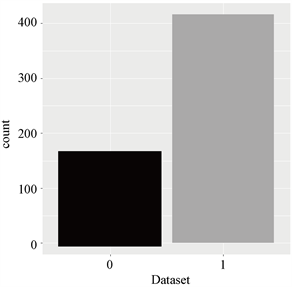Figure 2. No disease and number of patients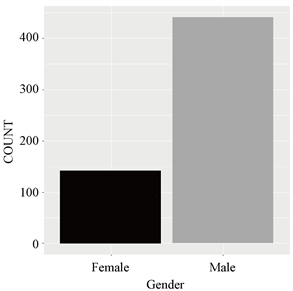Figure 3. Number of sick men and women

4.2. 数据分析

4.3. 深度对分类准确性的影响

4.4. 森林中树木数量对分类准确性的影响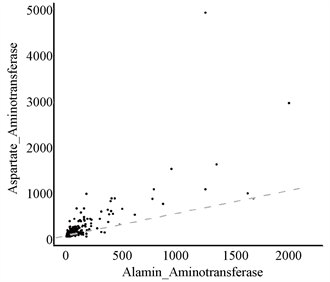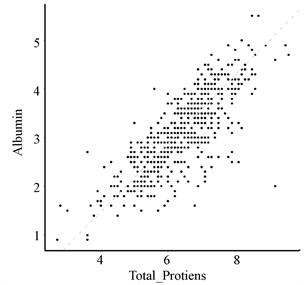Figure 4. Scatter plots of Total_Bilirubin & Direct_Bilirubin, Alamine_Aminotransferase & Aspartate_Aminotransferase, Total_Protiens & AlbuminFigure 5. Heat map visualization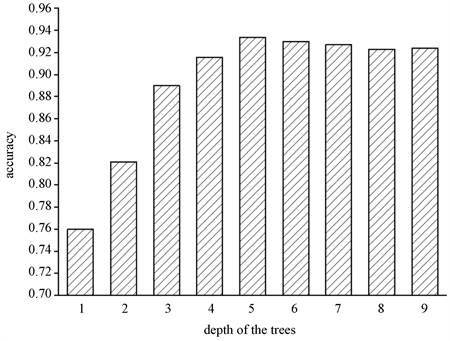Figure 6. Classification accuracy corresponding to different depths in random foreststFigure 7. Relationship between OOB error rate and number of decision trees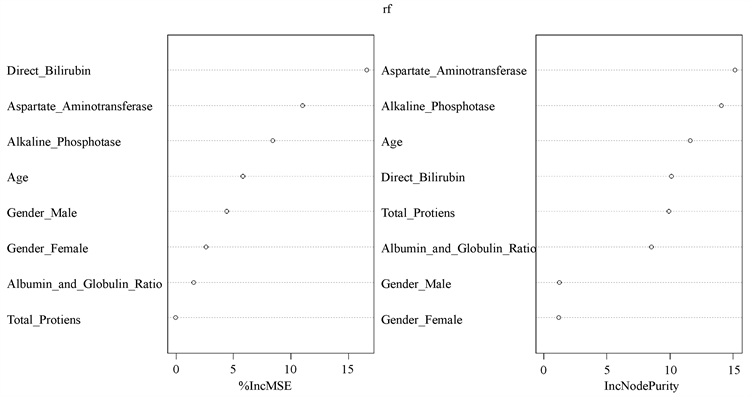Figure 8. Visualization graph for input variable importance measures

4.5. 与其他分类器对比

$accuracy=\frac{\text{TP}+\text{TN}}{\text{TP}+\text{TN}+\text{FP}+\text{FN}}$ (5)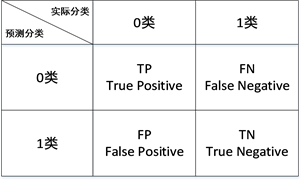Figure 9. Two-class confusion matrix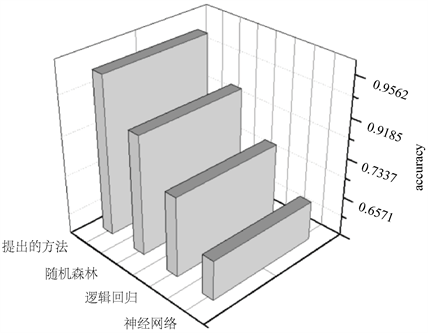Figure 10. Accuracy of running four classifiers

5. 结语

Diagnostic Prediction of Liver Cirrhosis Based on Improved Random Forest[J]. 计算机科学与应用, 2019, 09(10): 1928-1938. https://doi.org/10.12677/CSA.2019.910216

1. 1. 左颖婷. 遗传算法BP神经网络在肝硬化分期诊断中的应用[D]: [硕士学位论文]. 太原: 山西医科大学, 2017.

2. 2. 孙振球. 医学统计学[M]. 北京: 人民卫生出版社, 2007: 333-341.

3. 3. 张宁, 周双男, 宫嫚, 等. Fi-broScan评价复方鳖甲软肝片抗纤维化的疗效[J]. 临床肝胆病杂志, 2013, 29(10): 760-763.

4. 4. 窦智丽. 肝炎肝硬化患者症状、证候要素与瞬时弹性成像检测值的相关性研究[D]: [硕士学位论文]. 北京: 北京中医药大学, 2019.

5. 5. 范宏. 贝叶斯在医疗诊断系统中的应用研究[D]: [硕士学位论文]. 成都: 电子科技大学, 2013.

6. 6. 霍东雪, 刘辉, 尚振宏, 等. 一种异构集成学习的儿科疾病诊断方法研究[J]. 计算机应用与软件, 2018, 35(6): 54-57+157.

7. 7. Singh, B.K., Verma, K. and Thoke, A.S. (2015) A Dual Feature Selection Approach for Classification of Breast Tumors in Ultrasound Images Using ANN and SVM. Artificial Intelligent Systems & Machine Learning, 7, 78-84.

8. 8. Singh, B.K., Verma, K. and Thoke, A.S. (2016) Fuzzy Cluster Based Neural Classifier for Classifying Breast Tumors in Ultrasound Images. Expert Systems with Applications, 66, 114-123. https://doi.org/10.1016/j.eswa.2016.09.006

9. 9. Bikesh, K.S. (2019) Determining Relevant Biomarkers for Predic-tion of Breast Cancer Using Anthropometric and Clinical Features: A Comparative Investigation in Machine Learning Paradigm. Biocybernetics and Biomedical Engineering, 39, 393-409. https://doi.org/10.1016/j.bbe.2019.03.001

10. 10. Angshuman, P. and Dipti, P.M. (2019) Reinforced Quasi-Random Forest. Pattern Recognition, 94, 13-24.https://doi.org/10.1016/j.patcog.2019.05.013

11. 11. Feng, W., Dauphin, G., Huang, W.J., Quan, Y. and Liao, W. (2019) New Margin-Based Subsampling Iterative Technique in Modified Random Forests for Classification. Knowledge-Based Systems, 182, Article ID: 104845. https://doi.org/10.1016/j.knosys.2019.07.016
http://www.sciencedirect.com/science/article/pii/S095070511930320X

12. 12. 王宇燕. 基于决策树集成学习的癌症存活性预测分析[D]: [硕士学位论文]. 大连: 大连理工大学, 2018.

13. 13. Bedi, J. and Toshniwal, D. (2019) PP-NFR: An Improved Hybrid Learning Approach for Porosity Prediction from Seismic Attributes Using Non-Linear Feature Reduction. Journal of Applied Geophysics, 166, 22-32.https://doi.org/10.1016/j.jappgeo.2019.04.015

14. 14. Breiman, L. (2011) Random Forests. Machine Learning, 45, 5-32. https://doi.org/10.1023/A:1010933404324

15. 15. Cutler, A., Cutler, D.R. and Stevens, J.R. (2012) Random-forests. In: Zhang, C. and Ma, Y., Eds., Ensemble Machine Learning, Springer, Boston, MA, 157-175. https://doi.org/10.1007/978-1-4419-9326-7_5

16. 16. Nadi, A. and Moradi, H. (2019) Increasing the Views and Re-ducing the Depth in Random Forest. Expert Systems with Applications, 138, 112801. https://doi.org/10.1016/j.eswa.2019.07.018

17. 17. 普事业, 刘三阳, 白艺光. 网络拓扑特征的不平衡数据分类[J/OL]. 智能系统学报, 2019(5): 1-9. http://kns.cnki.net/kcms/detail/23.1538.TP.20190527.0921.002.html, 2019-08-11.

18. NOTES

*通讯作者。